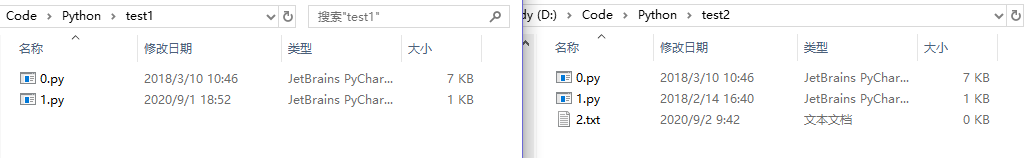# 用 Python 对比两个目录下的内容，并生成 Json 文件

### 三、代码

import hashlib
import os
import json

dic = {}

def getHash(f):
"""
获取文件哈希值
f：文件
"""
hash = hashlib.md5()
while(line):
hash.update(line)
return hash.hexdigest()

def IsHashEqual(f1,f2):
"""
比较哈希值是否相同
f1：第一个文件
f2：第二个文件
"""
str1 = getHash(f1)
str2 = getHash(f2)
return str1 == str2

def CountFiles(path1, path2):
"""
比较文件
"""
path_1, path_2 = [], []
file_dir1, file_dir2 = [], []
# 获取 path1 路径下的所有文件
for file in os.listdir(path1):
file_dir1.append(file)
# print(file)
tmp_path1 = path1 + str(file)
# print(path1)
path_1.append(tmp_path1)

# 获取 path2 路径下的所有文件
for file in os.listdir(path2):
file_dir2.append(file)
# print(file)
tmp_path2 = path2 + str(file)
# print(path2)
path_2.append(tmp_path2)

len1, len2 = len(path_1), len(path_2)

for i in range(min(len1, len2)):
file1 = open(path_1[i], "rb")
file2 = open(path_2[i], "rb")
res = IsHashEqual(file1, file2)
dic[file_dir1[i]] = res
# print(dic)

# 两个路径下的文件数量不同
if len1 < len2:
for i in range(len1, len2):
dic[file_dir2[i]] = False
elif len1 > len2:
for i in range(len2, len1):
dic[file_dir1[i]] = False

# 写入 json 文件
js = json.dumps(dic)
with open('test_data.json', 'w') as json_file:
json_file.write(js)

if __name__ == '__main__':
# f1 = open("D:/Code/Python/test1/0.py","rb")
# f2 = open("D:/Code/Python/test2/0.py","rb")
# print(IsHashEqual(f1,f2))
path1 = "D:/Code/Python/test1/"
path2 = "D:/Code/Python/test2/"
CountFiles(path1, path2)
print(dic)


### 四、结果

test1test2 两个文件夹下的内容如下图所示：{"0.py": true, "1.py": false, "2.txt": false}


### 五、需要改进的地方

• 只考虑文件目录一层结构，如果目录下面还有二级目录，怎么继续判断二级目录下的内容是否相同？
• 是否可以同步更新两个目录下的内容，保证一致性？

### 六、参考

Python判断两个文件是否相同与两个文本进行相同项筛选的方法

Python对象转换为json的方法步骤

【Python】字典内容写入json文件